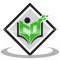## Write 'True' or 'False' and justify your answer in each of the following:If the height of a tower and the distance of the point of observation from its foot, both, are increased by $10 \%$, then the angle of elevation of its top remains unchanged.Updated on 10-Oct-2022 13:28:58

## Write 'True' or 'False' and justify your answer in each of the following:$(\tan \theta+2)(2 \tan \theta+1)=5 \tan \theta+\sec ^{2} \theta$.Updated on 10-Oct-2022 13:28:58
Given:$(\tan \theta+2)(2 \tan \theta+1)=5 \tan \theta+\sec ^{2} \theta$.To do:We have to find whether the given statement is true or false.Solution:We know that,$\sec ^{2} \theta-\tan ^{2} \theta=1$Therefore,$(\tan \theta+2)(2 \tan \theta+1)=2 \tan ^{2} \theta+4 \tan \theta+\tan \theta+2 $$=2(\sec ^{2} \theta-1)+5 \tan \theta+2$$=2 \sec ^{2} \theta+5 \tan \theta$The given statement is true.

## Write 'True' or 'False' and justify your answer in each of the following:If $\cos \mathrm{A}+\cos ^{2} \mathrm{~A}=1$, then $\sin ^{2} \mathrm{~A}+\sin ^{4} \mathrm{~A}=1$.Updated on 10-Oct-2022 13:28:58

## Write 'True' or 'False' and justify your answer in each of the following:The value of the expression $\left(\sin 80^{\circ}-\cos 80^{\circ}\right)$ is negative.Updated on 10-Oct-2022 13:28:58
Given:The value of the expression $\left(\sin 80^{\circ}-\cos 80^{\circ}\right)$ is negative.To do:We have to find whether the given statement is true or false.Solution:We know that,$\sin \theta$ is increasing when, \$0^{\circ}
Previous 1 ... 3 4 5 6 7 ... 775 Next